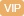| APP | 校园号 | 客服 客服热线：400-863-9889

400-863-9889【考点】因数与倍数【专题】约数倍数应用题．
【分析】按从左到右的序计算算；

【解答】解：25÷
 5 6
×
 6 5

01×
 4 5
-
 4 5

=1×
 1 2

=
 9 13

=×16+
 5 8
×16
=
 9 14
÷
 13 14

=
 3 4
×
 9 13
×
 4 3

=
 9 13

=
 4 5
×（101-）
=2×
 6 5
×
 6 5

=8×
 1 18
×（
 4 5
×
 5 8

=
 4 5
×10
=3×
 6 5

=7；
（4+
 5 8
）×6
=
 9 14
×
 14 13

 4 5
18×
 5 8
×
 1 18

80．
【点评】合运算的键是抓住运顺，正确按算顺序计，适当利用运定律简算．

0/0